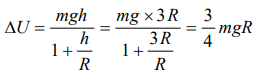## Gravitation Questions and Answers Part-12

1. The mass of the earth is $6.00\times 10^{24}kg$   and that of the moon is $7.40\times 10^{22}kg$   . The constant of gravitation $G=6.67\times 10^{-11}N-m^{2}\diagup kg^{2}$     . The potential energy of the system is $-7.79\times 10^{28}$   joules. The mean distance between the earth and moon is
a) $3.80\times 10^{8}metres$
b) $3.37\times 10^{6}metres$
c) $7.60\times 10^{4}metres$
d) $1.90\times 10^{2}metres$

Explanation:2. The change in potential energy, when a body of mass m is raised to a height nR from the earth's surface is (R = Radius of earth)
a) $mgR \frac{n}{n-1}$
b) nmgR
c) $mgR \frac{n^{2}}{n^{2}+1}$
d) $mgR \frac{n}{n+1}$

Explanation:3. The masses and radii of the earth and moon are $M_{1}, R_{1}$   and $M_{2}, R_{2}$   respectively. Their centres are distance d apart. The minimum velocity with which a particle of mass m should be projected from a point midway between their centres so that it escapes to infinity is
a) $2\sqrt{\frac{G}{d}\left(M_{1}+M_{2}\right)}$
b) $2\sqrt{\frac{2G}{d}\left(M_{1}+M_{2}\right)}$
c) $2\sqrt{\frac{Gm}{d}\left(M_{1}+M_{2}\right)}$
d) $2\sqrt{\frac{Gm\left(M_{1}+M_{2}\right)}{d\left(R_{1}+R_{2}\right)}}$

Explanation:4. If mass of earth is M, radius is R and gravitational constant is G, then work done to take 1 kg mass from earth surface to infinity will be
a) $\sqrt{\frac{Gm}{2R}}$
b) $\frac{GM}{R}$
c) $\sqrt{\frac{2Gm}{R}}$
d) $\frac{Gm}{2R}$

Explanation: Potential energy of the 1 kg mass which is placed at the earth surface = $\frac{-GM}{R}$
its potential energy at infinite = 0
Work done = change in potential energy = $\frac{GM}{R}$

5.A rocket is launched with velocity 10 km/s. If radius of earth is R, then maximum height attained by it will be
a) 2R
b) 3R
c) 4R
d) 5R

Explanation: 4R

6. There are two bodies of masses 100 kg and 10000 kg separated by a distance 1 m. At what distance from the smaller body, the intensity of gravitational field will be zero
a)$\frac{1}{9}m$
b) $\frac{1}{10}m$
c) $\frac{1}{11}m$
d) $\frac{10}{11}m$

Explanation:7. What is the intensity of gravitational field of the centre of a spherical shell
a) $Gm\diagup r^{2}$
b) g
c) Zero
d) none of these

Explanation: Zero

8. The gravitational potential energy of a body of mass ‘m’ at the earth’s surface $-mgR_{e}$ . Its gravitational potential energy at a height Re from the earth’s surface will be (Here Re is the radius of the earth)
a) $-2mgR_{e}$
b) $2mgR_{e}$
c) $\frac{1}{2}mgR_{e}$
d) $-\frac{1}{2}mgR_{e}$

Explanation:9. Escape velocity of a body of 1 kg mass on a planet is 100 m/sec. Gravitational Potential energy of the body at the Planet is
a) – 5000 J
b) – 1000 J
c) – 2400 J
d) 5000 J10. A body of mass m is placed on the earth’s surface. It is taken from the earth’s surface to a height $h=3R$ . The change in gravitational potential energy of the body is
a) $\frac{2}{3}mgR$
b) $\frac{3}{4}mgR$
c) $\frac{mgR}{2}$
d) $\frac{mgR}{4}$